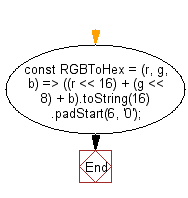# JavaScript: Convert the values of RGB components to a color code

## JavaScript fundamental (ES6 Syntax): Exercise-252 with Solution

Write a JavaScript program to convert the values of RGB components to a color code.

• Convert given RGB parameters to hexadecimal string using bitwise left-shift operator (<<) and Number.prototype.toString(16).

Sample Solution:

JavaScript Code:

``````//#Source https://bit.ly/2neWfJ2
const RGBToHex = (r, g, b) => ((r << 16) + (g << 8) + b).toString(16).padStart(6, '0');
console.log(RGBToHex(255, 165, 1));
console.log(RGBToHex(255, 255, 1));
```
```

Sample Output:

```ffa501
ffff01
```

Pictorial Presentation:Flowchart:Live Demo:

See the Pen javascript-basic-exercise-252-1 by w3resource (@w3resource) on CodePen.

Improve this sample solution and post your code through Disqus

What is the difficulty level of this exercise?

Test your Programming skills with w3resource's quiz.

﻿